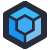Gregor needed to download a network driver. Upon clicking the link, a "captcha" appeared, presumably to prevent hotlinking to the driver files. It wasn't a real, image-based captcha, but a simple "here's some characters, type them into the box".

The code which popped up was "S i u x q F b j NaN 4". He hit the "new code" button, and got "T o A 0 J V s L NaN a". In fact, "NaN" showed up in the penultimate position in every code.

Curious, Gregor pulled up the debugger to see how the captcha was generated.

``````function Captcha(){
var alpha = new Array('A','B','C','D','E','F','G','H','I','J','K','L','M','N','O','P','Q','R','S','T','U','V','W','X','Y','Z',
'a','b','c','d','e','f','g','h','i','j','k','l','m','n','o','p','q','r','s','t','u','v','w','x','y','z',
'0','1','2','3','4','5','6','7','8','9');
var i;
for (i=0;i<20;i++){
var a = alpha[Math.floor(Math.random() * alpha.length)];
var b = alpha[Math.floor(Math.random() * alpha.length)];
var c = alpha[Math.floor(Math.random() * alpha.length)];
var d = alpha[Math.floor(Math.random() * alpha.length)];
var e = alpha[Math.floor(Math.random() * alpha.length)];
var f = alpha[Math.floor(Math.random() * alpha.length)];
var g = alpha[Math.floor(Math.random() * alpha.length)];
var h = alpha[Math.floor(Math.random() * alpha.length)];
var i = alpha[Math.floor(Math.random() * alpha.length)];
var j = alpha[Math.floor(Math.random() * alpha.length)];
}
var code = a + ' ' + b + ' ' + ' ' + c + ' ' + d + ' ' + e + ' '+ f + ' ' + g + ' ' + h + ' ' + i + ' ' + j;
Well, obviously not. The loop almost always executes only once. The variable `i` is not simply the loop variable, but also one of the variables holding the captcha characters. If it's set to a letter, `i<20` is false, and the loop breaks. If it's set to a number, the loop executes again (meaning that if you're really unlucky, the loop could possibly execute for a very long time).
It's a bit embarrassing to admit, but I had to think for a minute to figure out why `i` was `NaN`, and not just whatever letter it got assigned. Then I remembered- a for loop is just a while loop, and the increment step is executed at the end of the loop. Calling `++` on a string assigns `NaN` to the variable.
In Gregor's case, typing the captcha, `NaN` and all, into the input box worked just fine, and he was able to download his drivers.[Advertisement] ProGet’s got you covered with security and access controls on your NuGet feeds. Learn more.